﻿ The the Cosmological Constant Problems Based on the Duality of Time Theory## Solving the Cosmological Constant Problems Based on the Duality of Time Theory

The following article is extracted from Chapter III of the Duality of Time book, and some of the details are discussed further in other chapters, as well as Volume III that is the Ultimate Symmetry.

Please also check the related problems of Dark Matter and Dark Energy.As we noted in section 3, in order to make the Universe static, Einstein first added the cosmological constant to his field equations so that the attraction of matter may balance the cosmological constant repulsion. This postulate was later discarded after Hubble’s discovery of the expansion of the Universe in 1929. Nevertheless, in 1998, after the discovery of the accelerating Universe from distant Type Ia supernovae, in addition to the data from the cosmic microwave background and large galaxy redshift surveys, astronomers were able to measure the evolution of the expansion rate of the Universe which lead to the current standard model of cosmology, the Lambda-Cold-Dark-Matter model: (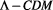) as we described in section 6.6.

However, galactic observations and large scale cosmology put an upper bound on the value of the cosmological constant in the range, which means that the vacuum energy density:, according to cosmological parameters from SDSS and WMAP, and the ratio between the energy density due to the cosmological constant and the critical density of the Universe is currently estimated at.

On the other hand, as early as 1960s, Quantum Field Theory predicted that quantum fluctuations would make huge contributions to the vacuum energy, much larger than the energy due to the cosmological constant which was known to be either zero or very small. The problem became critical after the development of inflationary models in the 1980s, since the cosmic inflation is driven by vacuum energy.

There are many problems associated with the cosmological constant. The observed acceleration of the expansion simply means that the cosmological constant is about 120 orders of magnitude smaller than the density of vacuum energy, the Planck density, that can be calculated from Quantum Field Theories. This is known as the problem of smallness. Moreover, being so small, why it just happens to have exactly the value that makes its density similar to the average matter density, which is called the coincidence problem.

In Quantum Field Theories, the vacuum energy density is due to the zero-point energy of quantized fields, which originates from the quantization of the simple harmonic oscillations of a particle with mass. The zero-point energy of the Klein-Gordon field is infinite, but a cutoff at Planck length is enforced, because it is generally believed that General Relativity does not hold for distances smaller than this length:, which corresponds to Planck energy: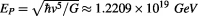.

The origin of vacuum energy density in Quantum Field Theories according to the Standard Model of particles is the electromagnetic, the weak and the strong interactions. From these estimations we get: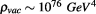. Comparing this theoretical value with the observed as we stated above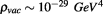, we find a 120 orders of magnitude discrepancy.

The smallness of the cosmological constant became a critical issue after the development of cosmic inflation in the 1980s, because the different inflationary scenarios are very sensitive to the actual value of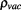. Many solutions have been suggested in this regard, including various modifications on either General Relativity or Quantum Field Theory or the way they are linked together.

The quintessence models suggest that the vacuum density may in fact be time-dependent, while other models involve attempts to quantize gravity, which Weinberg believe to be ‘the most promising’ although they usually involve mathematically ill-defined procedures. In the super-symmetric theory, because each particle has a super-partner, the fermion and boson contributions to the vacuum energy would cancel to zero. However, since we do not observe such super-partners in nature, so the super-symmetry must be broken. String theory has also failed to give a plausible solution. Since the problem really involves Quantum Gravity, Strings Theory should be the framework for addressing it. However, the cosmological constant problem has historically been a main obstacle of making Strings Theory more realistic. Finally, the proposal of an anthropic solution is based on the idea that our Universe is embedded in a larger multi-verse, so we happen to live in the right Universe whose cosmological constant is compatible with conditions for life forms to evolve. If the cosmological constant is positive and too large, the Universe will enter an expanding phase too early without the formation of galaxies. If it is negative and too large the Universe will collapse too fast.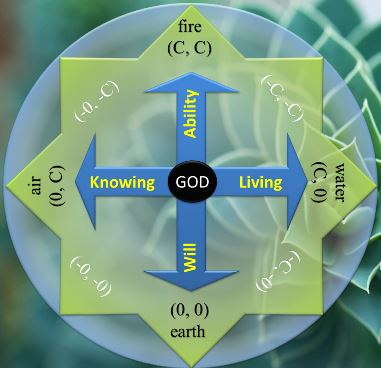Because the discrepancy is so huge, none of the above speculations came ever close to solving the puzzle. The discrepancy indicates that there is something substantially wrong in our understanding of quantum physical processes. The number of atoms in the visible universe is usually estimated at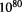, by considering that all atoms are Hydrogen atoms, and counting only the number of protons, which is called Eddington number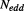. Taking the fact visible matter is only a small part of the universe and protons are made of quarks which could also have some fine structure, in addition to the number of electrons and other particles, we arrive to the conclusion that the discrepancy in the cosmological constant problem is still several orders of magnitudes larger than the number of all particles in the universe. It would not be strange, therefore, if we postulate that this huge physical multiplicity could be deceiving! Yet since we clearly observe multiplicity in our normal time level, the only way is to consider that the whole universe is made from only one ultimate, or metaphysical, elementary particle, that we call the Single Monad, that is multiplied in the inner level of time to create the whole physical universe.

According to the Duality of Time postulate, the huge discrepancy in the cosmological constant is diminished and even eliminated because the vacuum energy should be calculated from the average of all states, and not their collective summation as it is currently treated in QFT. This means that we should divide the vacuum energy density by the number of modes included in the unit volume. Since we take Planck length as the cutoff, this number is: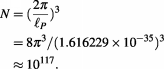(3.20)

This will reduce the discrepancy between the observed vacuum energy density and the prediction of QFT from 120 into only three orders of magnitudes. The remaining very small discrepancy could be now explained according to quintessence models, but a more accurate calculation is needed first because all the current methods to calculateare approximate. Also one possibility is that the actual cutoff which resembles the quanta of space could be a little smaller than Planck length.

Actually, according to the initial conclusions of this model that we discussed above, the quanta of space could potentially take any arbitrarily small value. This value would be larger in empty space and smaller in dense material, and thus much smaller in the early history of the universe or in black holes. That is why quintessence models would be promising in this regard, since they are formulated on scalar fields with time-dependent equation of state, while the cosmological constant postulate is characterized with fixed energy density, which means that the ratio of energy pressureto mass density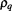is: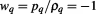, while this equation of state for quintessence varies with time. It was found in 2004 that the equation of state had possibly crossed the cosmological constant boundary from above to below. This no-go theorem is called the Quintom scenario and it requires at least two degrees of freedom for dark energy models.

### List of Some Major Problems Solved by the Duality of Time Theory

In the following articles, we will list some of the major unsolved problems in theoretical physics and describe them in brief, stating their potential solutions according to the Duality of Time Postulate. Although many of these problems will be simply eliminated according to the new genuinely-complex time-time geometry, a detailed theoretical and mathematical analysis is required in order to explain how these problems are settled. Therefore, some of the following brief suggested solutions may be speculative.

These articles are extracted from Chapter III of the Duality of Time book, and some of the details are discussed further in other chapters, as well as Volume III that is the Ultimate Symmetry. A more concise description is also published in Time Chest.

### The Duality of Time PostulateTo understand how all these major problems could be solved so easily, please study first the Duality of Time Postulate, that has been extracted from Chapter V of the Duality of Time book.

### Message from the Author:I have no doubt that this is the most significant discovery in the history of mathematics, physics and philosophy, ever!

This unique understanding of geometry will cause a paradigm shift in our knowledge of the fundamental nature of the cosmos and its corporeal and incorporeal structures.

Enjoy reading... , all the Best !

Mohamed bin Ali Haj Yousef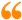The science of Time is a noble science, that reveals the secret of Eternity. Only the Elites of Sages may ever come to know this secret. It is called the First Age, or the Age of ages, from which time is emerging.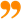Ibn al-Arabi [The Meccan Revelations: Volume I, page 156. - Trns. Mohamed Haj Yousef]

### Ibn al-Arabi Website:### The Meccan Revelations:### The Sun from the West:عربي Arabic

## Social Sharing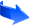Like Our Facebook Page:Like Our Facebook Page:The Moving Finger writes; and, having writ,... Moves on: nor all thy Piety nor Wit, shall lure it back to cancel half a Line. Nor all thy Tears wash out a Word of it.